$$\newcommand{\id}{\mathrm{id}}$$ $$\newcommand{\Span}{\mathrm{span}}$$ $$\newcommand{\kernel}{\mathrm{null}\,}$$ $$\newcommand{\range}{\mathrm{range}\,}$$ $$\newcommand{\RealPart}{\mathrm{Re}}$$ $$\newcommand{\ImaginaryPart}{\mathrm{Im}}$$ $$\newcommand{\Argument}{\mathrm{Arg}}$$ $$\newcommand{\norm}{\| #1 \|}$$ $$\newcommand{\inner}{\langle #1, #2 \rangle}$$ $$\newcommand{\Span}{\mathrm{span}}$$

# C: Geometric Formulas

$$\newcommand{\vecs}{\overset { \rightharpoonup} {\mathbf{#1}} }$$

$$\newcommand{\vecd}{\overset{-\!-\!\rightharpoonup}{\vphantom{a}\smash {#1}}}$$

### Table C1 - 2 Dimensions

Name Shape Formulas
Rectangle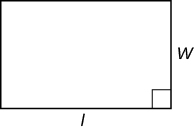Perimeter: P = $$2l + 2w$$

Area: A = $$lw$$

SquarePerimeter: P = 4s

Area: A = s2

TrianglePerimeter: P = a + b + c

Area: A = $$\frac{1}{2}$$bh

Sum of Angles: A + B + C = 180°

Right Triangle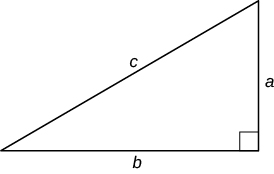Pythagorean Theorem: a2 + b2 = c2

Area: A = $$\frac{1}{2}$$ab

Circle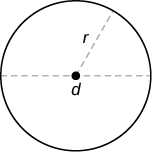Circumference: C = 2$$\pi$$r or C = $$\pi$$d

Area: A = $$\pi$$r2

ParallelogramPerimeter: P = 2a + 2b

Area: A = bh

TrapezoidPerimeter: P = a + b + c + B

Area: A = $$\frac{1}{2}$$(B + b)h

### Table C2 - 3 Dimensions

Name Shape Formulas
Rectangular Solid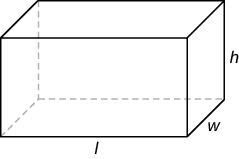Volume: V = $$lwh$$

Surface Area: SA = $$2lw + 2wh + 2hl$$

CubeVolume: V = s3

Surface Area: SA = 6s2

Cone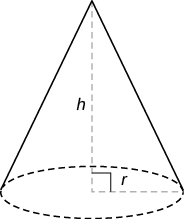Volume: V = $$\frac{1}{3} \pi r^{2} h$$

Surface Area: SA = $$\pi r^{2} + \pi r \sqrt{h^{2} + r^{2}}$$

SphereVolume: V = $$\frac{4}{3} \pi r^{3}$$

Surface Area: SA = $$4 \pi r^{2}$$

Right Circular CylinderVolume: V = $$\pi r^{2} h$$

Surface Area: SA = $$2 \pi r^{2} + 2 \pi rh$$